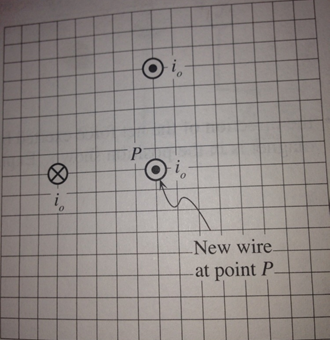# Problem: Shown at the right is a cross-sectional view of two long straight wires that are parallel to one another. One wire carries current io out of the page, the other carries an equal current into the page.Suppose that a third wire, carrying another current io out of the page, passes through point P.Draw a vector on the diagram to indicate the magnetic force, if any, exerted on the current in the new wire at P. If the magnitude of the force is zero, indicate explicitly. Explain your reasoning.

###### FREE Expert Solution

Magnetic force on a current-carrying wire:

$\overline{){\mathbf{F}}{\mathbf{=}}{\mathbf{B}}{\mathbf{i}}{\mathbf{L}}{\mathbf{s}}{\mathbf{i}}{\mathbf{n}}{\mathbf{\theta }}}$

The current and the magnetic field are perpendicular to each other.

F = BiL(sin90) = BiL###### Problem Details

Shown at the right is a cross-sectional view of two long straight wires that are parallel to one another. One wire carries current io out of the page, the other carries an equal current into the page.

Suppose that a third wire, carrying another current io out of the page, passes through point P.

Draw a vector on the diagram to indicate the magnetic force, if any, exerted on the current in the new wire at P. If the magnitude of the force is zero, indicate explicitly. Explain your reasoning.